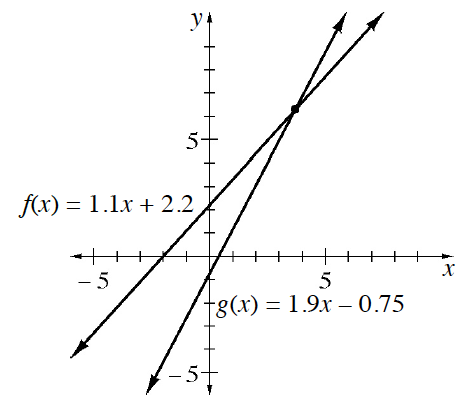### Home > GB8I > Chapter 6 Unit 7 > Lesson INT1: 6.2.3 > Problem6-73

6-73.

The graph below shows the lines $f(x)=1.1x+2.2$ and $g(x)=1.9x-0.75$. One combination of input and output values will satisfy both functions. How do you know this? Use the graph to estimate this combination of values. Then create a table of values for each function and use it to justify your answer.What is the point at which the two lines intersect?

A reasonably close estimate is $(3.5,6)$ based solely on the provided graph.